Author: Oscar Cronquist Article last updated on December 07, 2020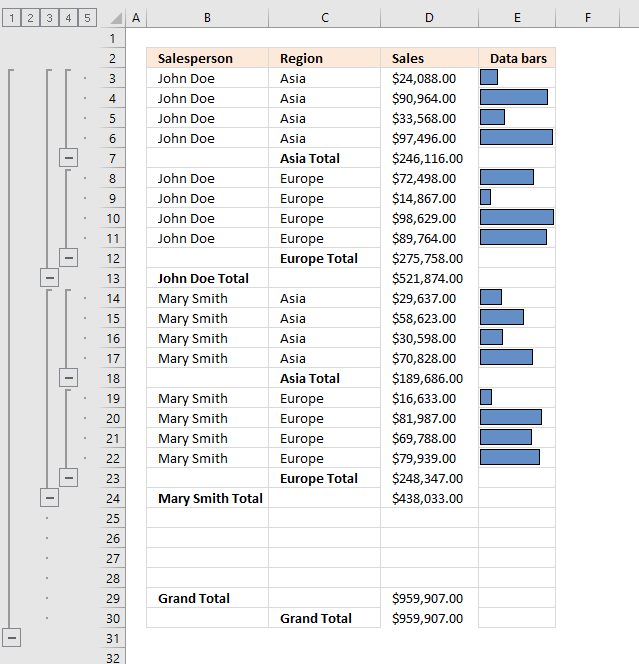The Subtotal feature lets you insert totals and grand totals automatically, this feature was added to Excel 2010 and is still around in later Excel versions. The image above demonstrates the Subtotal tool applied to cell range B2:E30.

The + and minus signs to the left of the row numbers let you expand and collapse rows respectively. This functionality lets you easily examine the values behind a specific subtotal that may interest you more than the others.

### Prepare data

Before you can use the Subtotal tool you need to prepare your data:

• Remove blank rows.
• Sort the data based on the column or columns you want to add subtotals to.

#### How to sort data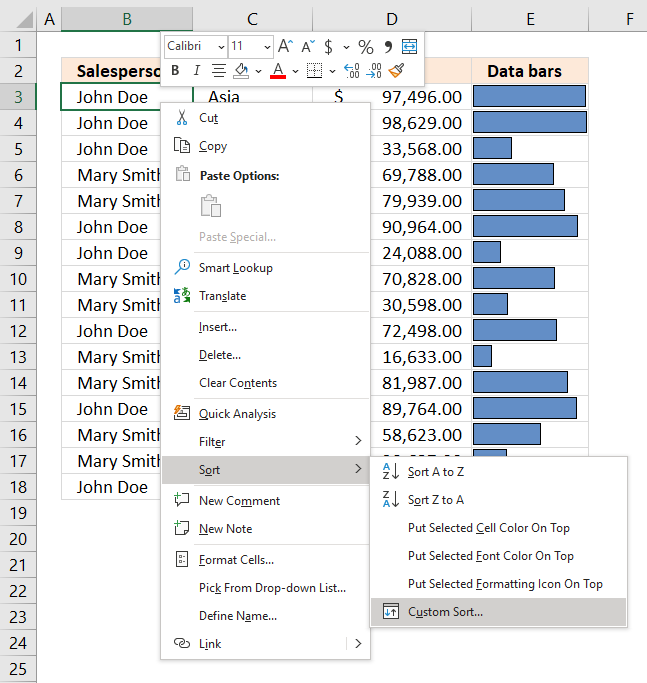1. Press with right mouse button on on any cell in your data set. A pop-up menu appears, see image above.
2. Press with mouse on "Sort".
3. Press with left mouse button on "Custom Sort...", a dialog box appears.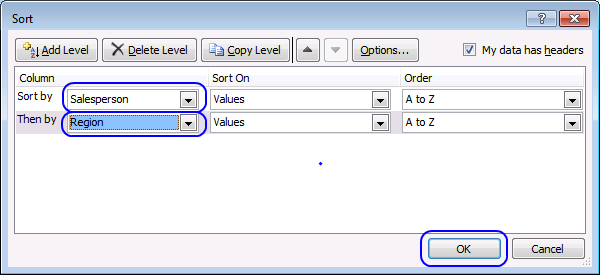4. Sort by "Salesperson"
6. Then by "Region"
7. Press with left mouse button on OK button.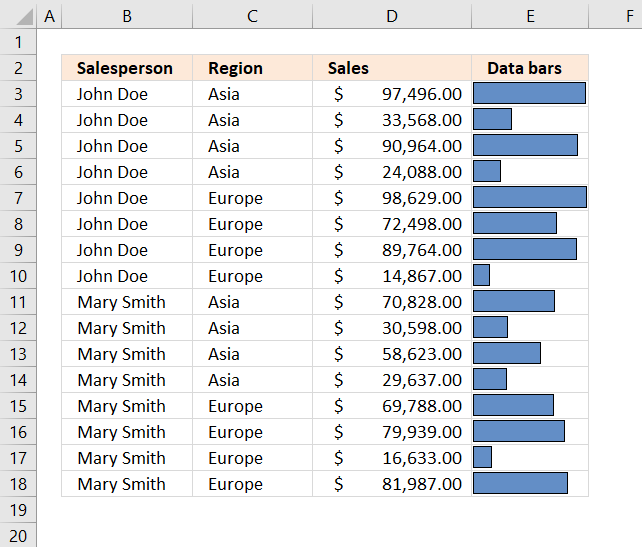The image above shows the data set sorted based on column B and then on column C.

Note, you can't insert subtotals to an Excel Table. This will grey out the "Subtotal" button.I recommend a Pivot Table if you want the functionality that the Excel Table has and the Subtotal feature.

### How to start the Subtotal tool?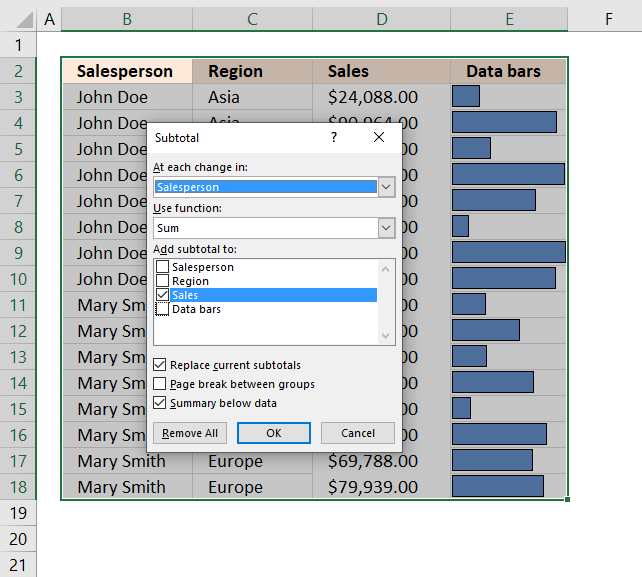Go to tab "Data" on the ribbon, press with left mouse button on the "Subtotal" button. A dialog box appears, see image above.

Disable the "Data Bars" checkbox and enable the "Sales" check box. Press with left mouse button on the OK button to apply settings and create subtotals.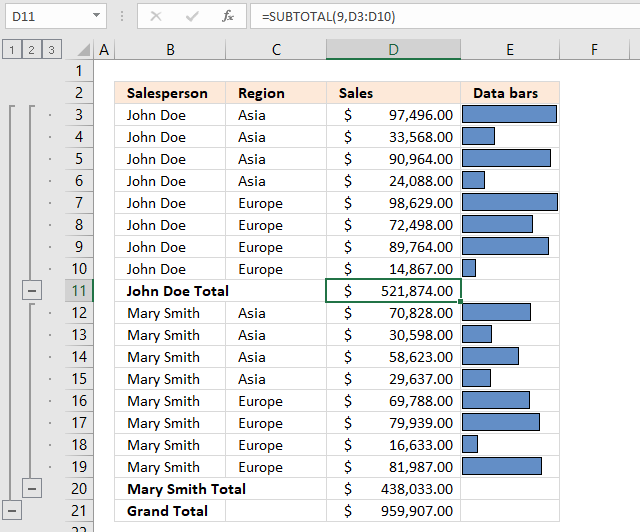The image above demonstrates subtotals in cell D11 and D20 created by the Subtotal tool. The formula bar shows that the Subtotal tool inserted SUBTOTAL functions in both cell D11 and D20.

The SUBTOTAL function has many arguments which I won't be demonstrating in this article, check out the SUBTOTAL function article if you want to learn more about them.

Press with left mouse button on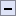symbol to collapse rows and press with left mouse button on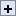symbol to expand rows. You can also expand or collapse all rows on a hierarchy level by pressing 1, 2 or 3: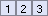These buttons are located to the left of the row numbers, see the image above or the animated image below.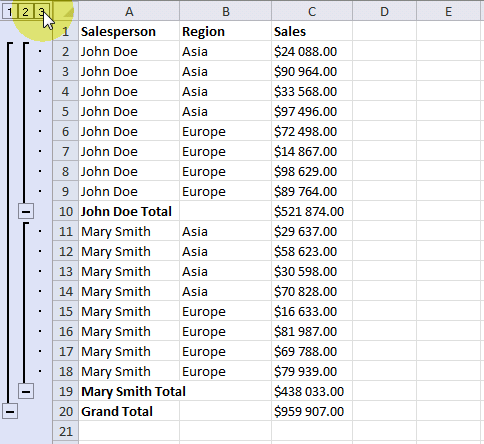### Subtotals on multiple levels

You can add more subtotals to your data list. We inserted subtotals before when a change occurred in column Salesperson, let's add a new subtotal for each change in column Region.

1. Select cell range A1:C20
2. Press with left mouse button on "Subtotal" button on tab "Data"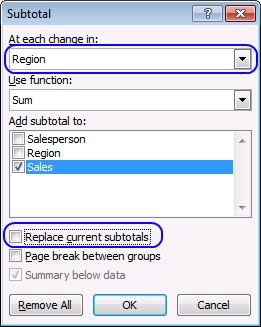3. Press with left mouse button on the drop-down menu below the "At each change in:"
4. Select Region
5. Clear checkbox "Replace current subtotals"
6. Press with left mouse button on OK button.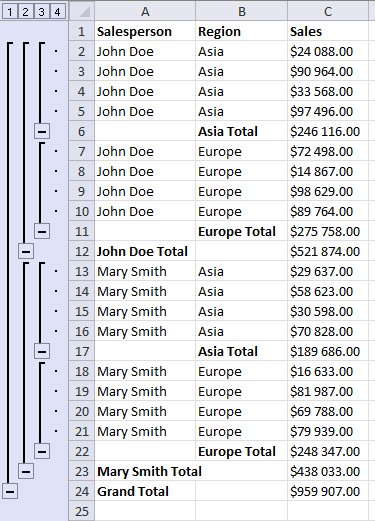### Dialog box settings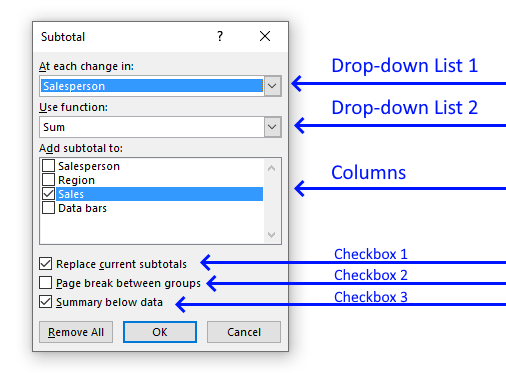#### At each change in

The first drop-down list lets you choose which column to use when determining where to insert subtotals to. When a value changes a new subtotal is inserted, this is why it is important to sort the values first.

#### Use function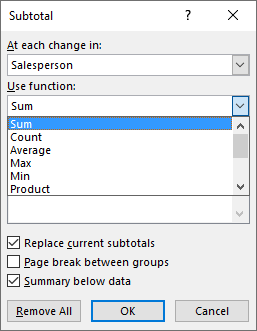The dialog box allows you to pick one function out of eleven functions, they are:

• ##### Sum - Calculates a total.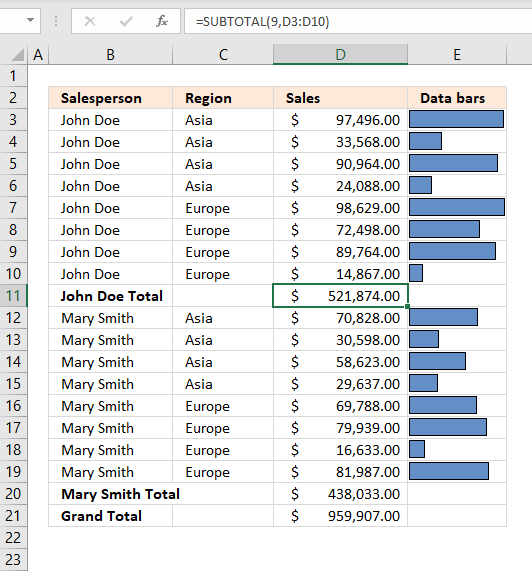Formula in cell D11:

=SUBTOTAL(9,D3:D10)

Number 9 represents the SUM function, it adds numbers and returns a total.
97496 + 33568 + 90964 + 24088 + 98629 + 72498 + 89764 + 14867 = 521874

• ##### Count - Counts the number of cells that are not empty.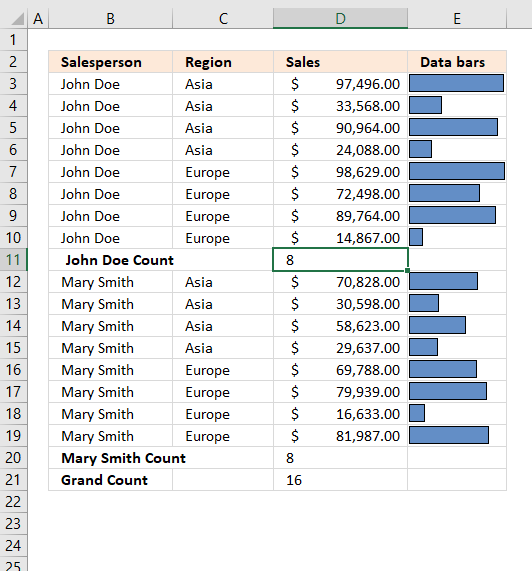Formula in cell D11:

=SUBTOTAL(3,D3:D10)

The first argument is 3 which represents the COUNTA function, it counts all cells that are not empty.
There are eight cells in cell range D3:D10 and they all non-empty. The formula returns 8 in cell D11.

• ##### Average - Returns the average (arithmetic mean) of the arguments.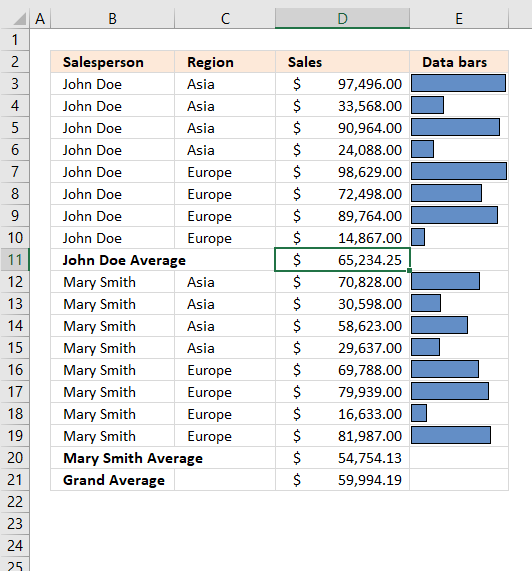Formula in cell D11:

=SUBTOTAL(1,D3:D10)

The first argument is 1, it represents the AVERAGE function. It adds all numbers and then divides by the total count of values.
97496 + 33568 + 90964 + 24088 + 98629 + 72498 + 89764 + 14867 = 521874
521874/8 = 65234.25

• ##### Max - Returns the largest number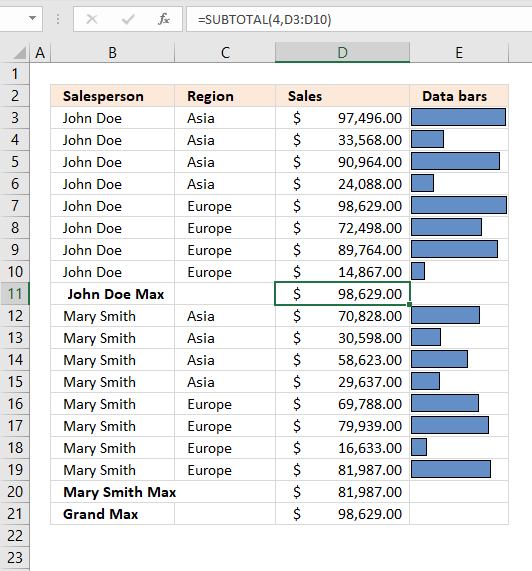Formula in cell D11:

=SUBTOTAL(4, D3:D10)

Cell range D3:D10 contains the following values: 97496, 33568, 90964, 24088, 98629, 72498, 89764 and 14867.
98629 is the largest one.

• ##### Min - Returns the smallest number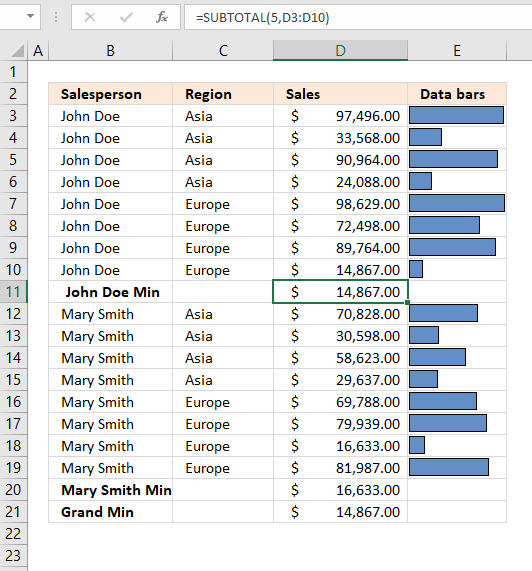Formula in cell D11:

=SUBTOTAL(5, D3:D10)

Cell range D3:D10 contains the following values: 97496, 33568, 90964, 24088, 98629, 72498, 89764 and 14867.
14867 is the smallest number.

• ##### Product - Multiplies numbers in cell range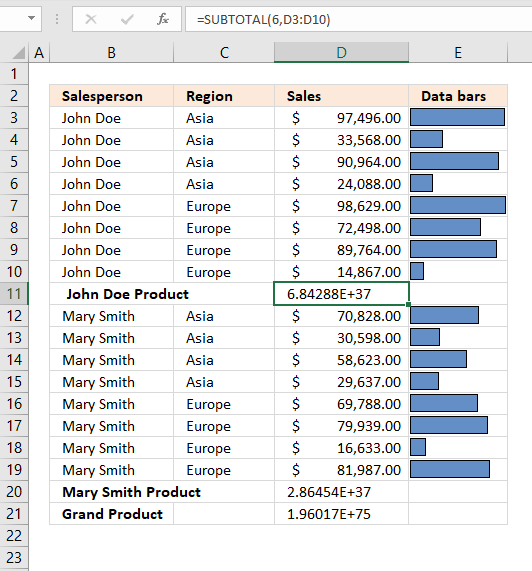Formula in cell D11:

=SUBTOTAL(6, D3:D10)

Cell range D3:D10 contains the following values: 97496, 33568, 90964, 24088, 98629, 72498, 89764 and 14867.
97496 * 33568 * 90964 * 24088 * 98629 * 72498 * 89764 * 14867 = 6.8428772708558E+37

• ##### Count numbers - Count the number of cells that contain numbersFormula in cell D11:

=SUBTOTAL(2, D3:D10)

There are eight cells in cell range D3:D10 and they all are numbers. The formula returns 8 in cell D11.

• ##### StdDev- Calculates the standard deviation based on a sample of the entire population.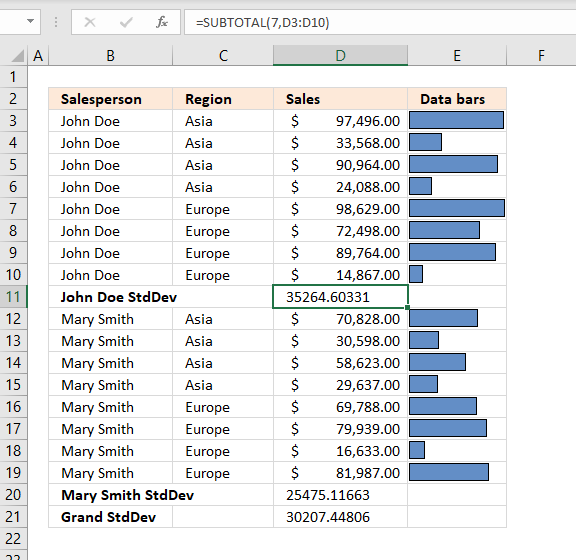Formula in cell D11:

=SUBTOTAL(7, D3:D10)

The first argument is 7 which represents the STDEV.S function, it was added to Excel 2010. The STDEV.S function returns the standard deviation based on a sample of the entire population.

The standard deviation shows how much the values differ from the mean value of the group, in other words, how they are distributed around the average value, or how spread out the numbers are.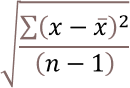x ̅ is the average.
n is how many values.

97496+33568+90964+24088+98629+72498+89764+14867 = 521874
521874/8 = 65234.25 is the average number.

(97496-65234.25)^2 + (33568-65234.25)^2 + (90964-65234.25)^2 + (24088-65234.25)^2 + (98629-65234.25)^2 + (72498-65234.25)^2 + (89764-65234.25)^2 + (14867-65234.25)^2

becomes

32261.75^2 + (-31666.25)^2 + 25729.75^2 + (-41146.25)^2 + 33394.75^2 + 7263.75^2 + 24529.75^2 + (-50367.25)^2

becomes

1040820513.0625 + 1002751389.0625 + 662020035.0625 + 1693013889.0625 + 1115209327.5625 + 52762064.0625 + 601708635.0625 +2536859872.5625

equals 8705145725.5.

8705145725.5/(8-1) = 1243592246.5

1243592246.5^(1/2) = 35264.6033084168

• ##### StdDevp - calculates the standard deviation based on the entire population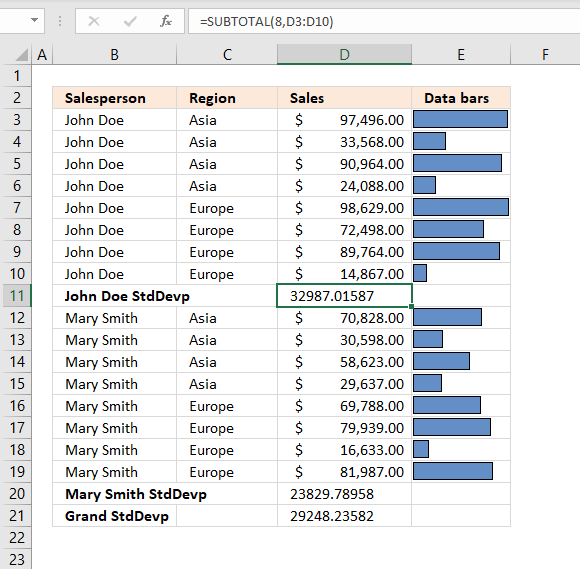Formula in cell D11:

=SUBTOTAL(8, D3:D10)

The STDEV.P function returns the standard deviation based on the entire population.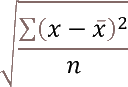x ̅ is the average.
n is how many values.

97496+33568+90964+24088+98629+72498+89764+14867 = 521874
521874/8 = 65234.25 is the average number.

(97496-65234.25)^2 + (33568-65234.25)^2 + (90964-65234.25)^2 + (24088-65234.25)^2 + (98629-65234.25)^2 + (72498-65234.25)^2 + (89764-65234.25)^2 + (14867-65234.25)^2

becomes

32261.75^2 + (-31666.25)^2 + 25729.75^2 + (-41146.25)^2 + 33394.75^2 + 7263.75^2 + 24529.75^2 + (-50367.25)^2

becomes

1040820513.0625 + 1002751389.0625 + 662020035.0625 + 1693013889.0625 + 1115209327.5625 + 52762064.0625 + 601708635.0625 +2536859872.5625

equals 8705145725.5.

8705145725.5/8 = 1088143215.6875

1088143215.6875^(1/2) = 32987.0158651476

• ##### Var - estimates variance based on a sample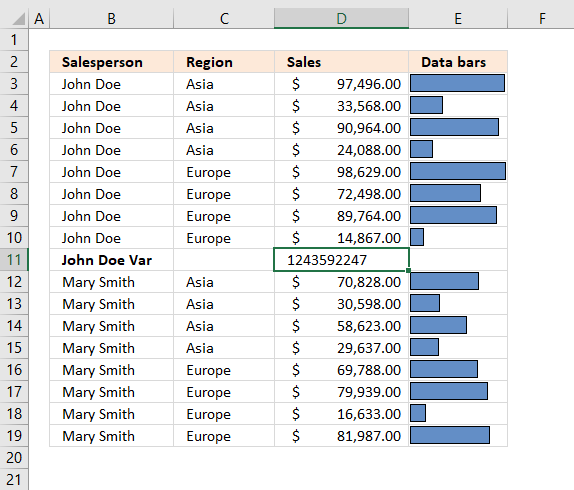Formula in cell D11:

=SUBTOTAL(10, D3:D10)

The VAR.S function returns the variance based on the entire population. Variance is the average of the squared differences from the mean.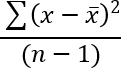x ̅ is the average.
n is how many values.

97496+33568+90964+24088+98629+72498+89764+14867 = 521874
521874/8 = 65234.25 is the average number.

(97496-65234.25)^2 + (33568-65234.25)^2 + (90964-65234.25)^2 + (24088-65234.25)^2 + (98629-65234.25)^2 + (72498-65234.25)^2 + (89764-65234.25)^2 + (14867-65234.25)^2

becomes

32261.75^2 + (-31666.25)^2 + 25729.75^2 + (-41146.25)^2 + 33394.75^2 + 7263.75^2 + 24529.75^2 + (-50367.25)^2

becomes

1040820513.0625 + 1002751389.0625 + 662020035.0625 + 1693013889.0625 + 1115209327.5625 + 52762064.0625 + 601708635.0625 +2536859872.5625

equals 8705145725.5.

8705145725.5/(8-1) = 1243592246.5

• ##### Varp - estimates variance based on population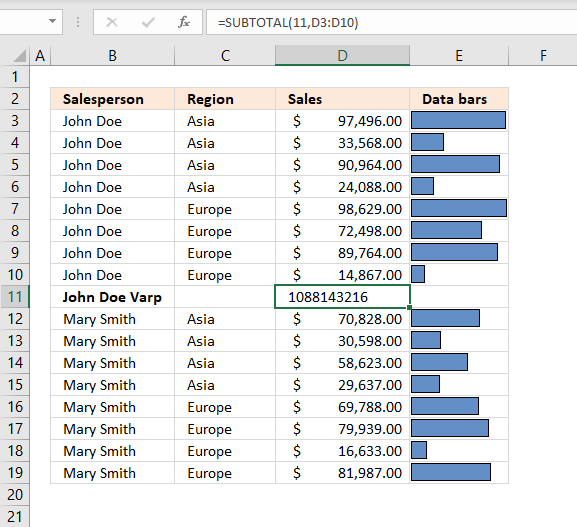Formula in cell D11:

=SUBTOTAL(10, D3:D10)

The VAR.P function returns the variance based on the entire population. Variance is the average of the squared differences from the mean.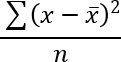x ̅ is the average.
n is how many values.

97496+33568+90964+24088+98629+72498+89764+14867 = 521874
521874/8 = 65234.25 is the average number.

(97496-65234.25)^2 + (33568-65234.25)^2 + (90964-65234.25)^2 + (24088-65234.25)^2 + (98629-65234.25)^2 + (72498-65234.25)^2 + (89764-65234.25)^2 + (14867-65234.25)^2

becomes

32261.75^2 + (-31666.25)^2 + 25729.75^2 + (-41146.25)^2 + 33394.75^2 + 7263.75^2 + 24529.75^2 + (-50367.25)^2

becomes

1040820513.0625 + 1002751389.0625 + 662020035.0625 + 1693013889.0625 + 1115209327.5625 + 52762064.0625 + 601708635.0625 +2536859872.5625

equals 8705145725.5.

8705145725.5/8 = 1088143215.6875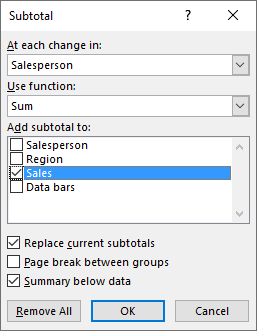This setting allows you to choose which columns to add subtotals to.

#### Replace current subtotalsThis checkbox lets you substitute current subtotals with new ones, if enabled.

#### Pagebreak between groupsThis checkbox, if enabled, lets you put each group in a new page.

#### Summary below dataThe last checkbox adds a summary below all groups, for example, if a total is calculated for each group then a grand total is calculated below all groups.

Now it is time to spice up your data table with some chart bars. Hold on, I am not going to insert a chart. Conditional Formatting is what we are going to use actually.

1. Create a new column and type =C2 in cell D2.
2. Copy cell D2 to the cells below.
3. Remove cells that link to subtotals and grand total.
4. Select cell range D2:D24.
5. Go to tab "Home" on the ribbon.
6. Press with left mouse button on "Conditional formatting" button.
7. Press with left mouse button on "Data Bars".
8. Press with left mouse button on "More Rules..."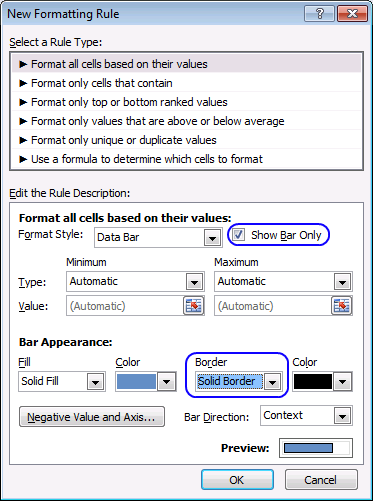9. Enable the checkbox "Show Bar Only".
10. I chose a "Solid Border" from the "Border" drop-down menu.
11. Press with left mouse button on OK button.

The Data Bars let you quickly compare numbers, finding outliers, or interesting statistics.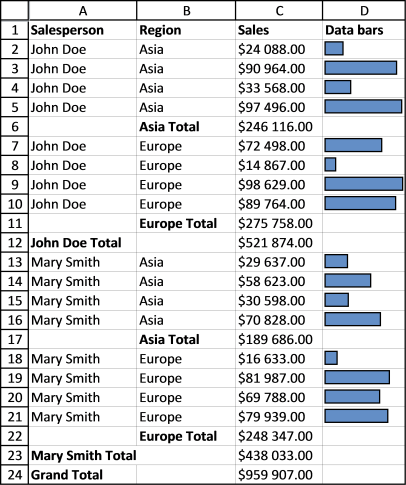### Remove all subtotals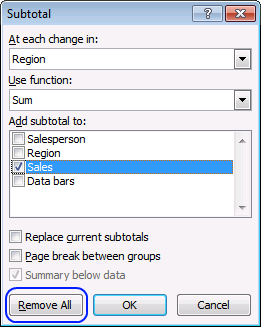You can easily remove all subtotals, select the cell range you want to remove subtotals from. Go to tab "Data", press with left mouse button on "Subtotal" button. Press with left mouse button on "Remove All" button.

### Build a Pivot Table/Pivot chart

A pivot table allows you to summarize a data table in many different ways, using different criteria. It is easy and you will be analyzing your data in no time. Pivot tables are subtotals on steroids.

I highly recommend reading this blog post: Analyze trends using pivot tables, there is also a quick introduction to pivot tables.

You can also compare data, year to year, month to month and whatever time frame you like.

### Get the Excel fileSubtotals-on-multiple-levels.xlsx